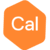# How to calculate the Average Cross Spectrum​Posts: 1edited October 2019
Hello,
I want to calculate the admittance function for two grids ( g(x,y) Bouger anomaly and h(x,y) topography). It is defined as:
(GH*)/(HH*)

where G(kx,ky) and H(kx,ky) are in the fourier space and () means average . The term (HH*)is the Radial Average Spectrum​ that can be calculated using MAGMAP, and (GH*) is the Average Cross Spectrum​, but i don't know how to calculate it. Can you help me ??

Thank
Tagged: